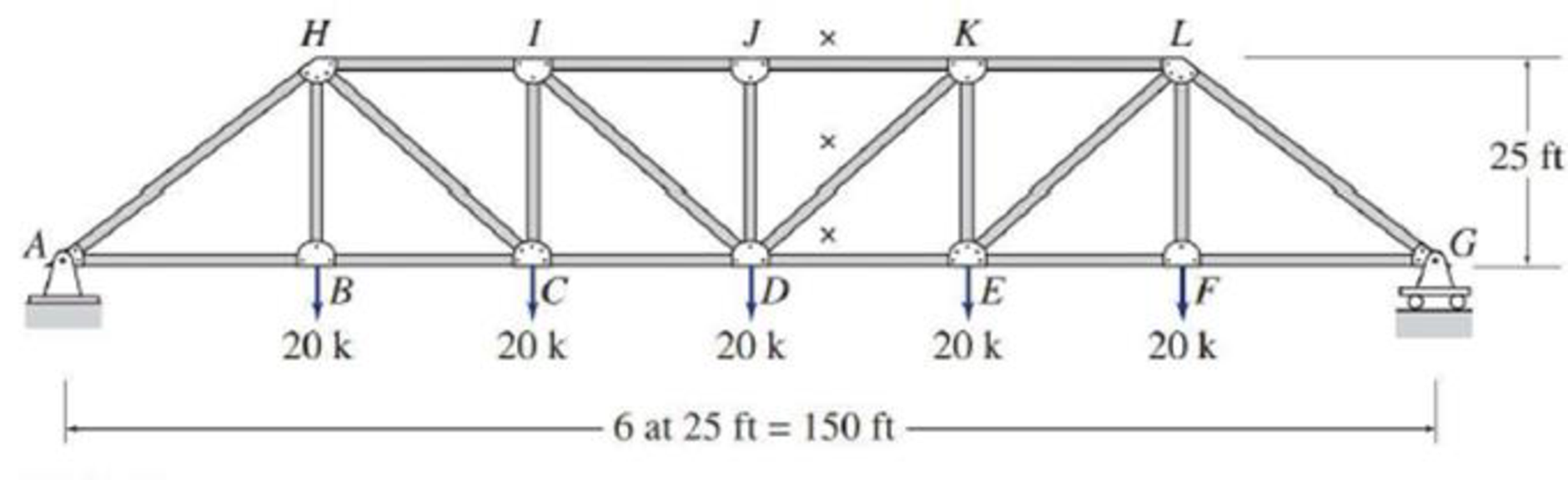# Determine the forces in the members identified by “x” of the truss shown by the method of sections. FIG. P4.33

#### Solutions

Chapter
Section
Chapter 4, Problem 33P
Textbook Problem
24 views

## Determine the forces in the members identified by “x” of the truss shown by the method of sections.FIG. P4.33

To determine

Find the forces in the members JK, DK, and DE of the truss by the method of sections.

### Explanation of Solution

Given information:

Apply the sign conventions for calculating reactions, forces and moments using the three equations of equilibrium as shown below.

• For summation of forces along x-direction is equal to zero (Fx=0), consider the forces acting towards right side as positive (+) and the forces acting towards left side as negative ().
• For summation of forces along y-direction is equal to zero (Fy=0), consider the upward force as positive (+) and the downward force as negative ().
• For summation of moment about a point is equal to zero (Matapoint=0), consider the clockwise moment as negative and the counter clockwise moment as positive.

Method of sections:

The negative value of force in any member indicates compression (C) and the positive value of force in any member indicates Tension (T).

The Figure of the truss is shown.

The members identified by “x” are JK, DK, and DE.

Consider the forces in the members JK, DK, and DE are denoted by FJK, FDK, and FDE.

Calculation:

Show the free body diagram of the truss as shown in Figure 1.

Refer Figure 1.

Consider the horizontal and vertical reaction at A are denoted by Ax and Ay.

Consider the vertical reaction at G is denoted by Gy.

Ay=Gy=(20+20+20+20+202)=50k

Find the angle (θ) made by the member DK with the horizontal as follows:

tanθ=EKDEtanθ=2525θ=tan1(1)θ=45°

Consider a section a-a passing through JK, DK, and DE.

Show the portion of the truss to the right of the truss as shown in Figure 2.

Refer Figure 1 and Figure 2.

For Equilibrium of forces,

Take the sum of all vertical forces in y direction as zero.

Fy=0FDK×sinθ2020+50=0FDK×sin45°+10=0FDK×sin45°=10

FDK=10sin45°FDK=14

### Still sussing out bartleby?

Check out a sample textbook solution.

See a sample solution

#### The Solution to Your Study Problems

Bartleby provides explanations to thousands of textbook problems written by our experts, many with advanced degrees!

Get Started

Find more solutions based on key concepts
The cash flow given in Problem 20.18 is to be replaced by an equivalent cash flow with equal amounts (X) at the...

Engineering Fundamentals: An Introduction to Engineering (MindTap Course List)

What is a vulnerability scanner? How is it used to improve security?

Principles of Information Security (MindTap Course List)

State the purpose of a DNS server.

Enhanced Discovering Computers 2017 (Shelly Cashman Series) (MindTap Course List)

Convert 5 megawatts of power into BTU/hr, ft-lbs/s, and kJ/hr.

Fundamentals of Chemical Engineering Thermodynamics (MindTap Course List)

List two types of canned cycles besides roughing turning and finish turning.

Precision Machining Technology (MindTap Course List)

Why must eye protection be worn at all times in the welding shop?

Welding: Principles and Applications (MindTap Course List)

If your motherboard supports ECC DDR3 memory, can you substitute non-ECC DDR3 memory?

A+ Guide to Hardware (Standalone Book) (MindTap Course List)